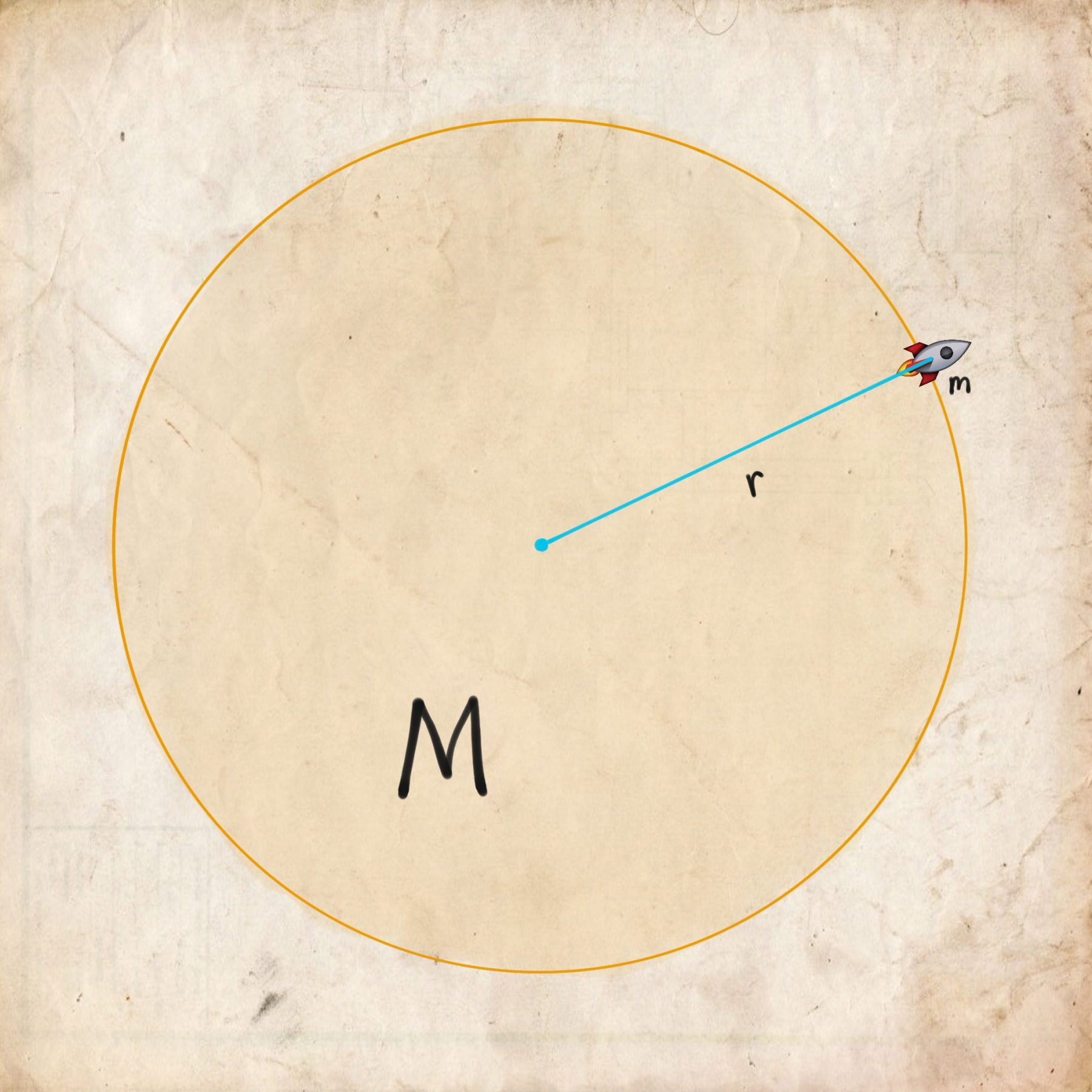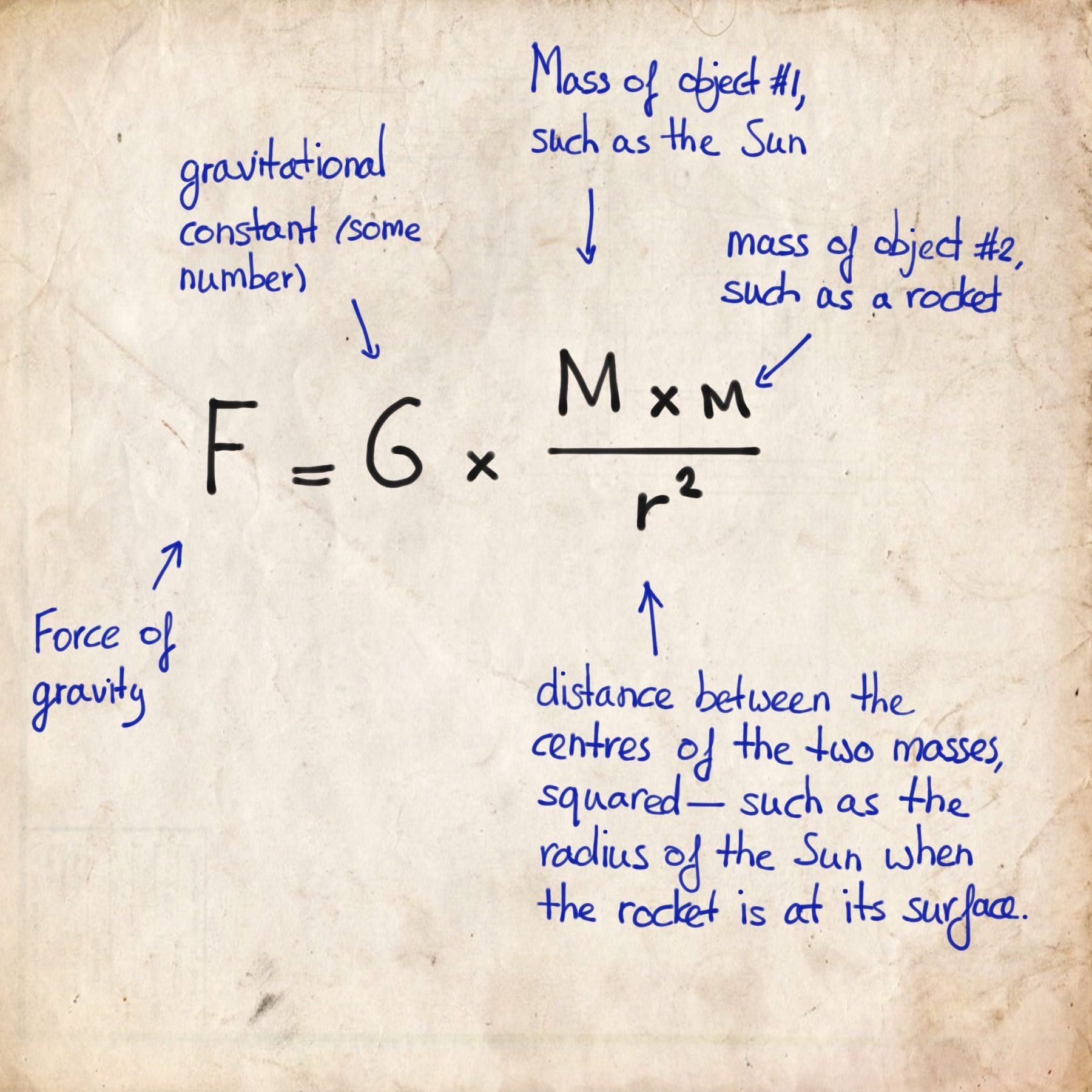# Just a minute: what is a black hole?

• 11
Shares

Much like any question in the vain of ‘what is (…love…)’, for which mathematical, physical, molecular, biological, psychological, philosophical, literary, and artistic approaches could be employed, here too, are several ways to approximate the answer to the question ‘What is a black hole?’

Let’s take the notion of escape velocity, which is the minimum speed (in a specific direction) needed for an object to break loose of the gravitational domination of a massive body. Larger gravity means that you have to fly faster to get off the planet.

Instead of a planet, let’s pretend we have a rocket on the surface of the Sun. Why the Sun, you might ask. Rest assured, we’ll definitely get to that. In the sketch below you see the situation at hand.Turns out, the larger the Sun’s mass, the stronger its gravitational influence on the rocket on its surface. Sounds obvious enough, right? In the sketch, the Sun’s mass is symbolised by ‘big $M$’. Of course, the rocket has a mass too, so this is denoted by ‘small m’. Lastly, the distance between the centre of the Sun(’s mass) and that of the rocket plays a big role. The larger the distance, the weaker the gravitational influence. This distance is denoted by the letter $r$. This obviously also means, the smaller the distance, the stronger the gravitational influence.

Assuming the mass of the rocket (‘small m’) stays constant, we say that the gravitational influence is proportional to $M$ and inversely proportional to $r$. We can now begin to describe a mathematical relationship between the gravitational influence, $M$, and $r$. We can write:

\begin{equation*} \text{gravitational influence} \propto \frac{M}{r}. \end{equation*}

This weird $\propto$-sign means ‘is proportional to’. The fraction $\frac{M}{r}$ means that, if $M$ grows bigger, the division grows bigger. If $r$ grows bigger, the division shrinks smaller. Let’s just fill in some numbers to see how this works. Suppose, $M = 600$ and $r = 5$.

\begin{equation*} \text{gravitational influence} \propto \frac{600}{5} = 120. \end{equation*}

Let’s make $M$ six times bigger: $M = 3600$. We get

\begin{equation*} \text{gravitational influence} \propto \frac{3600}{5} = 720. \end{equation*}

Not surprisingly, the division becomes six times larger too. If we make $r$ smaller, say $r=2$, the result becomes even larger:

\begin{equation*} \text{gravitational influence} \propto \frac{3600}{2} = 1800. \end{equation*}

So, what would happen if—in a thought experiment—we would add more mass $M$ to our Sun? Indeed, the gravitational influence on the rocket would become larger. In turn, the rocket would have to fly faster in order to leave the Sun.

What would happen if—in a further thought experiment—we would not just add more mass $M$ to our Sun but also shrink its radius $r$, so that it becomes a small, very dense ball of stuff? Indeed, the gravitational influence would become even larger, so, the rocket would have to fly even faster.

### Schwarzschild

The real formula that Newton came up with for the gravitational influence, which we call Newton’s law of universal gravitation, is a little different from what we have used up until now, and goes as follows:Of course, Einstein came up with an even more accurate set of formulas, but for our purpose, we won’t be using them as Newton’s law works just as well, in this case.

John Michell, an 18th-century, English philosopher and clergyman, basically wondered if the ratio between mass $M$ and distance $r$ could lead to a gravitational influence so big that the required speed for a rocket to fly off to the stars would exceed the speed of light. He wasn’t sure if anything with that much mass and such short a radius could ever exist, but if so, then, in theory, ‘dark stars’ could exist.

When some stars reach the end of their lives, they become supernovae. They explode their outer shells into space, while the inner shells of matter move inwards. This means that the surface quickly shrinks towards the centre of mass. This means that things, such as rockets, can get closer to the centre of mass while experiencing the gravitational influence of all that mass underneath it.

Given the amount of the star’s imploding mass $M$, at some point, there will be a distance $r$ around it where the gravitational influence is so large, that the minimum speed for a rocket to escape it will have to be larger than the speed of light.

It was Karl Schwarzschild, a German physicist who found this distance using Albert Einstein’s equations of general relativity (the more accurate set of formulas compared to Newton’s).

Given a certain mass, the Schwarzschild radius is the distance from the centre of mass below which the magnitude of the escape velocity is larger than the speed of light. And so, this part of the universe will be black, whence no one returns. Every point around the centre of this mass as described by the Schwarzschild radius, forms what we call the event horizon.

A black hole is thus a region in the universe where the gravitational influence is so large that nothing, not even light, can escape it. Or, to put it a bit more technically, it is a region of spacetime where every possible future leads to its singularity. We might explain the latter in another article, in the future.

### Event Horizon Telescope

A vast array of radio observatories and telescope facilities around the world basically turned our entire planet into one big telescope, called the Event Horizon Telescope.

On Wednesday, 10 April 2019, at 15:00 CET, the first photo of a supermassive black hole in the middle of a galaxy called Messier 87 was presented. Later, a photo of the black hole in our own Milky Way, called Sagittarius A*, will be expected.

The observations may test Einstein’s general relativity yet again. Perhaps more on that in another article.

We highly recommend watching the recording of the live stream of the presentation of the results.

We have been focussing on non-rotating black holes. The physical models for a rotating black holes differ to some degree, but not significantly for the scope of this article.

Featured image: the image of the supermassive black hole Messier 87. Credit: EHT Collaboration